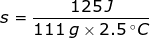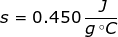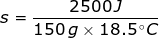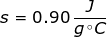# Specific Heat Capacity Formula

Specific Heat Capacity Formula

The specific heat capacity of a substance is the amount of heat required to raise one gram of the substance by one degree Celsius. Water, for example, has a specific heat capacity of 4.18. This means to heat one gram of water by one degree Celsius, it would require 4.18 joules of energy.s = specific heat capacity (sometimes represented by the letter c, or Cs)

q = heat

m = mass

Δ T = change in temperature

Specific Heat Capacity Formula Questions:

1. What is the specific heat capacity of iron if it takes 125 J of heat to raise 111 grams by 2.5 degrees Celsius?2. What is the specific heat capacity of aluminum if it takes 2500 J to raise 150 grams from 10°C to 28.5°C?

In this problem the change in temperature must be determined by taking the final temperature (Tf) minus the initial temperature (Ti).

Δ T = Tf - Ti

Δ T = 28.5°C – 10°C

Δ T = 18.5°C

Continue by solving the equation for specific heat capacity.Related Links: Heat Transfer Formula Specific Heat Formula